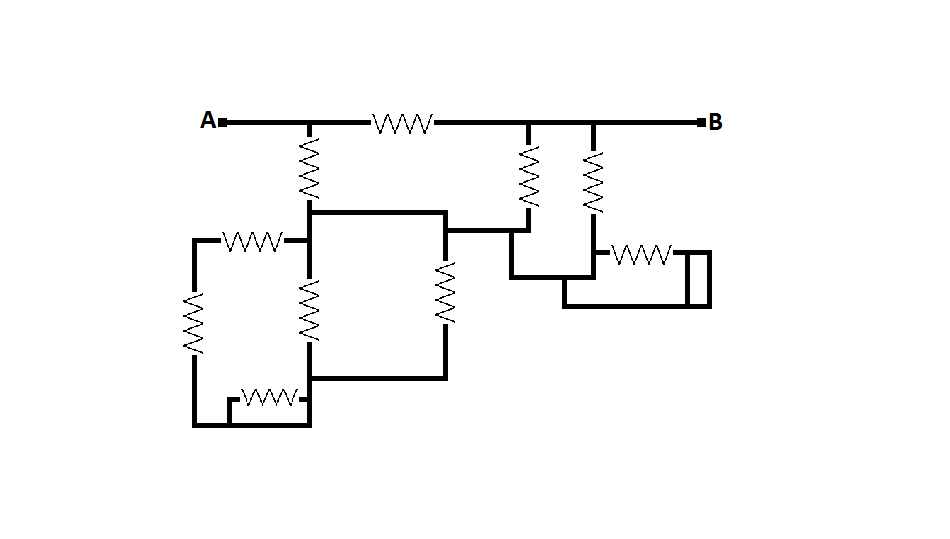# Net Resistance Problem!Find the net resistance between points $A$ and $B$ shown in the figure, given the value of resistance of each individual resistor is $1 \Omega$.

×